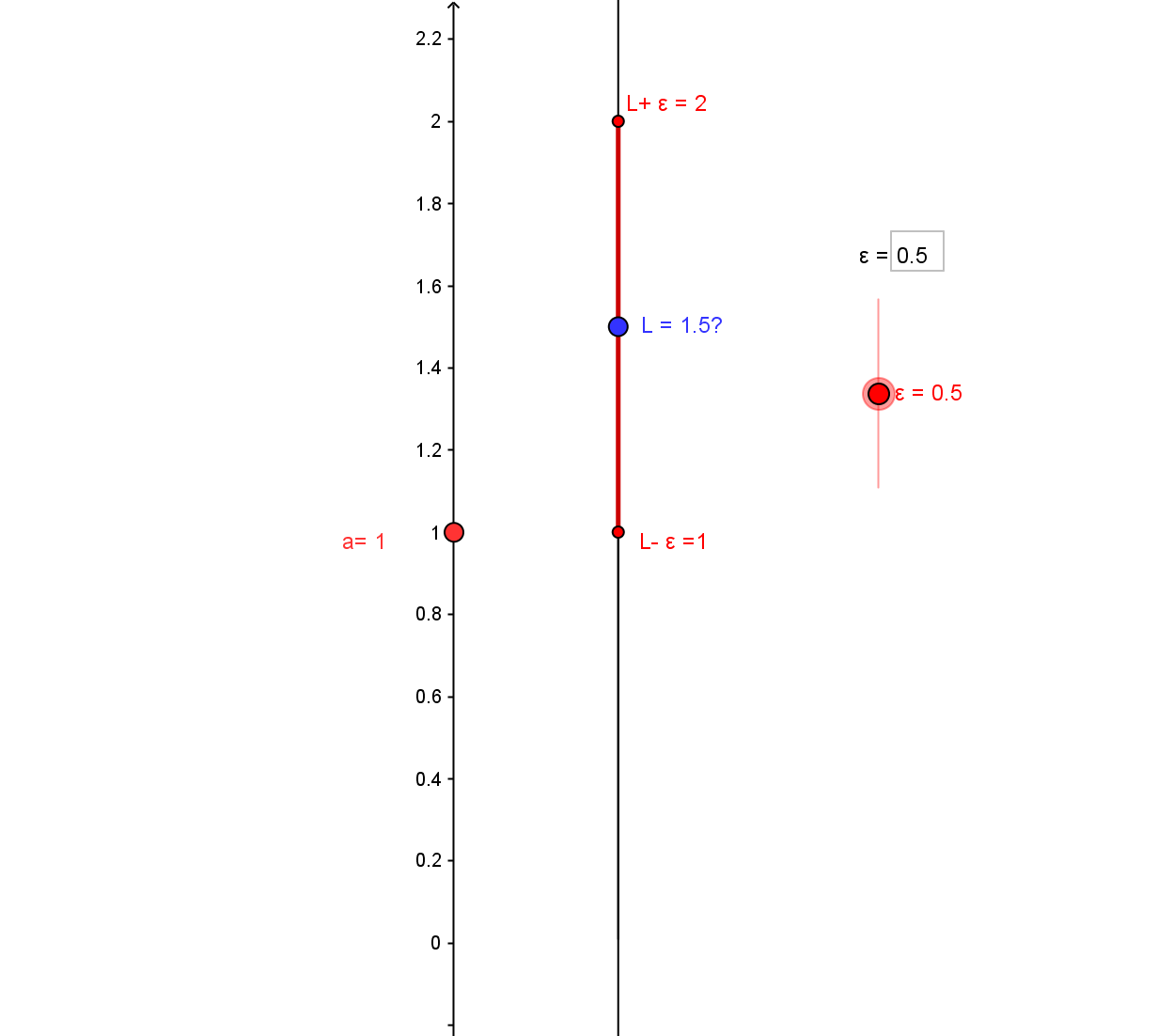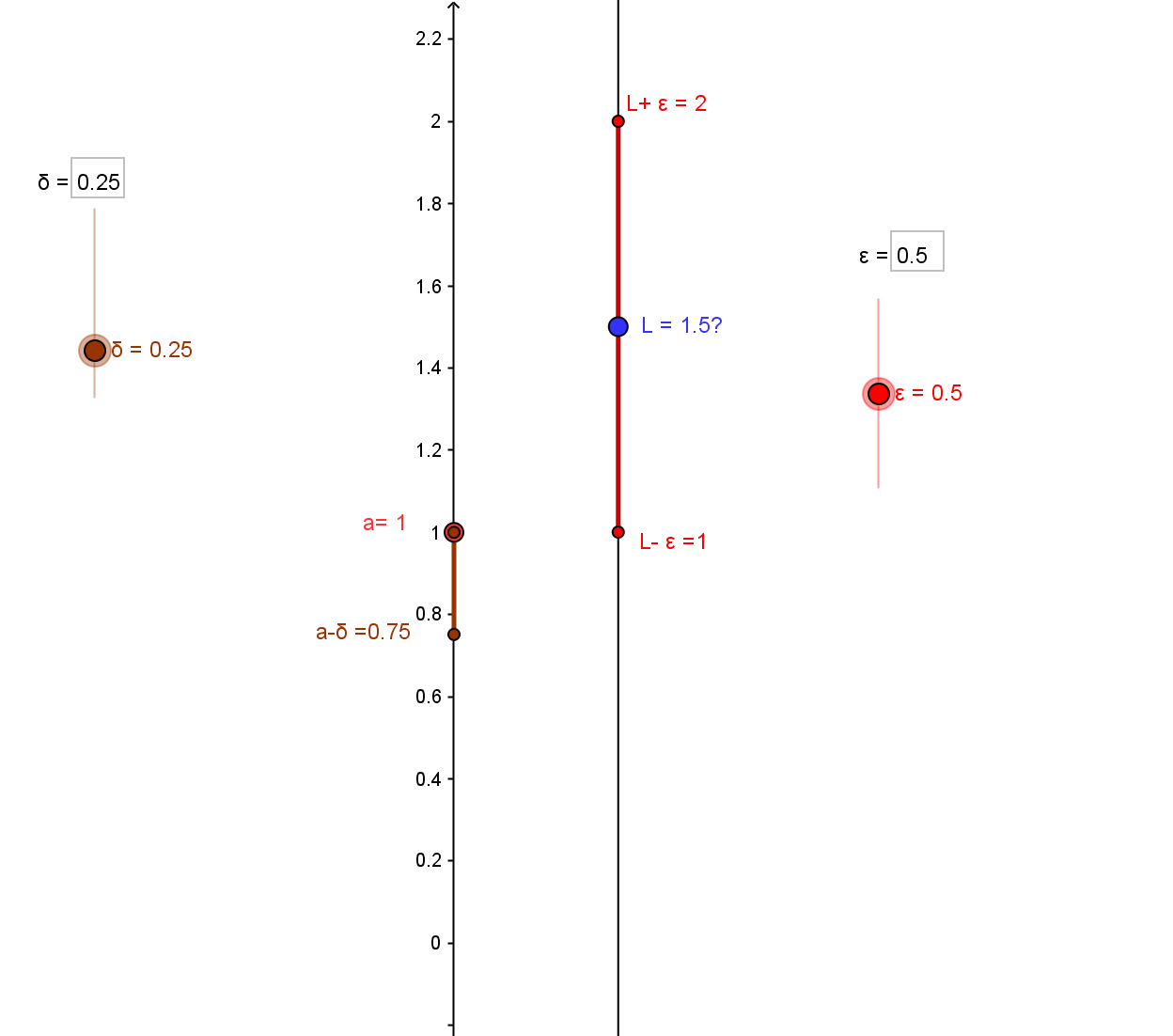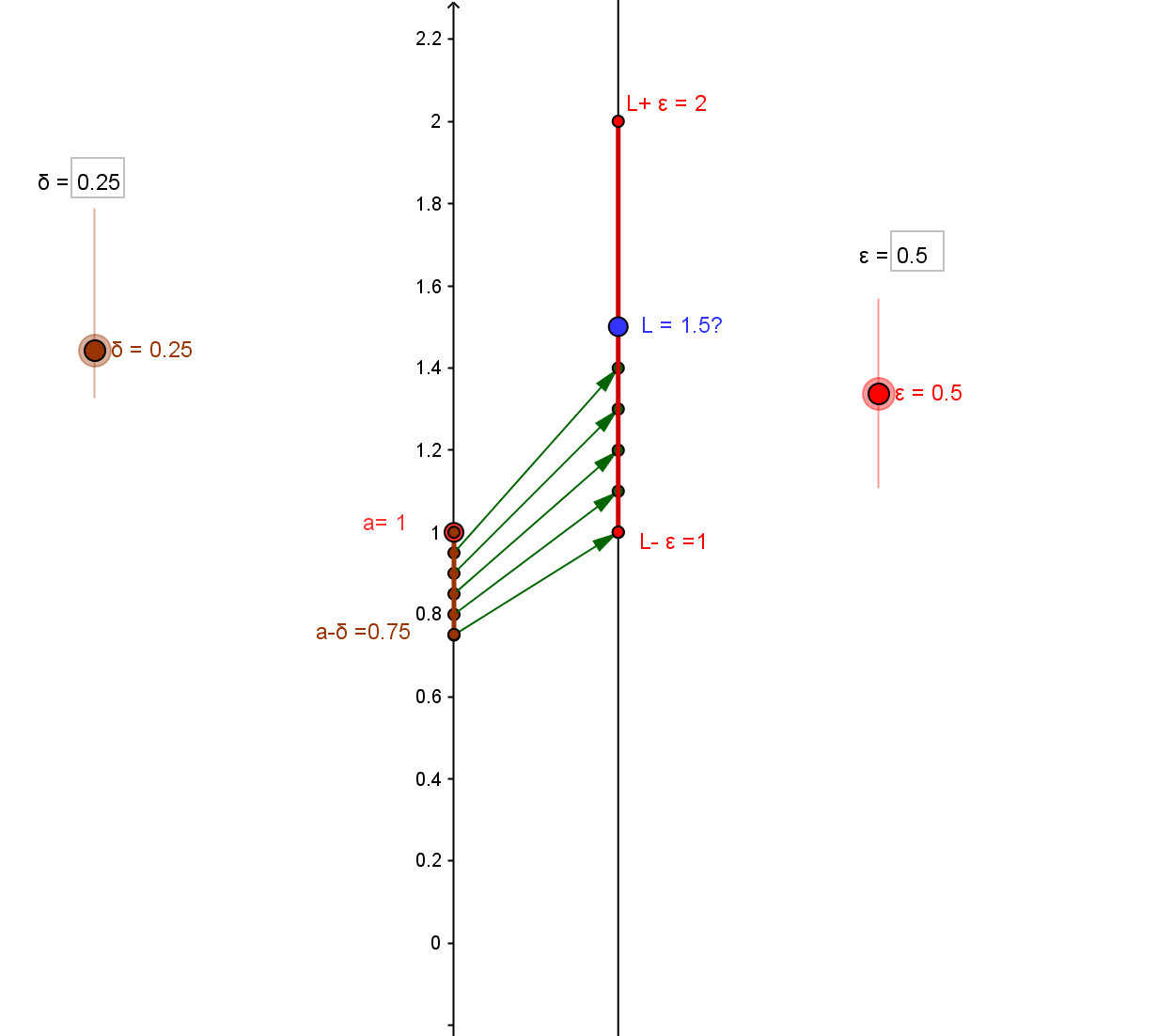Definition of One Sided Limit: CCD.DOSL.
A number $L$ is called the right side limit of $f$ as $x$ approaches $a$ from above, ($x \to a^+$), denoted $\lim_{x \to a^+} f(x) = L$ or $\lim_{x \downarrow a} f(x) = L$, if when $f$ is defined for all $x > a$ in an open interval containing $a$, the values $f(x)$ approach $L$, ($f(x) \to L$).
The limit, $L$, if such a number exists, is interpreted traditionally as...
1. The number that is the best estimate for values of $f(x)$ when $x$ is a number close to and greater than $a$;
2. The position that an object approaches at times close to $a$, when moving on a line with its position at time $x > a$ determined by $f(x)$;
3. On the graph of the function $f$, the second coordinate of a point that would fill in a hole in the graph of $f$ when considered omitting any points on the graph with first coordinate less than $a$.
The formal definition for $L$ to be called the limit says: Given any $\epsilon > 0$, there is a number $\delta >0$ with the property that if $a < x < a + \delta$, then $L-\epsilon < f(x)< L + \epsilon$.
This condition is also expressed:
Given any $\epsilon > 0$, there is a number $\delta >0$ with the property that if $0<x-a<\delta$, then $|f(x)-L|< \epsilon$.

A similar definition can be given for a number $L$ to be called the left side limit of $f$ as $x$ approaches $a$ from below, ($x \to a^-$), denoted $\lim_{x \to a^-} f(x) = L$ or $\lim_{x \uparrow a} f(x) = L$, namely, if when $f$ is defined for all $x < a$ in an open interval containing $a$, the values $f(x)$ approach $L$, ($f(x) \to L$).

Visualizing the definition of limit number with mapping diagrams provides visual support to understand the information contained in this number for the function values for $x$ near $a$, i.e., $x \approx a$.

To illustrate the operation of the definition  of  $\lim_{x \to a^-} f(x) = L$ with a mapping diagram, consider an example function $f(x)=2x-\frac12$ with $a=1$, $L=1.5$ and $\epsilon = 0.5$.
Since in the definition the values $f(x)$ will be compared with $L$, we begin by visualizing $L$ on the target axis of the diagram, as well as the interval
$(L-\epsilon , L + \epsilon)$.The choice of $\delta$, here we'll use $\delta =0.25$, is the essential feature of the definition, being made after the selection of $\epsilon$ and controlling the numbers $x$ to which the function is to be applied.
So the interval determined by $\delta=0.25$ for the left side limit is visualized on the domain axis of the diagram with the interval $(a-\delta, a)$.After the choice of $\delta$ the task is to show that any $x$ in the interval $(a-\delta, a)$, must have $f(x) \in (L-\epsilon , L + \epsilon)$. Using the arrows to visualize a sampling of relevant $x$ and related $f(x)$ values gives a sense of what must hold true for $\epsilon = 0.5$.But this quality must work not just
for the chosen $\epsilon$, but this ability to find an appropriate value for $\delta >0$ must be possible for any $\epsilon>0$.

A dynamic visualization of the definition of
$\lim_{x \to a^+} f(x) = L$ is found in CCD.DLCV, Dynamic visualization of the definitions of limit and continuity.